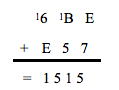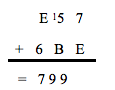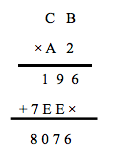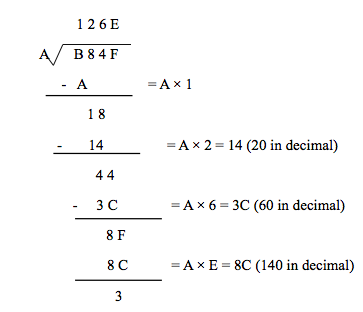# Hex Calculator

 Enter Number 1 Operation Addition (+) Subtraction (-) Multiply (x) Division (/) Enter Number 2

### OUTPUT

 Output

The Hex Calculator is used to perform addition, subtraction, multiplication and division on two hexadecimal numbers.

A hexadecimal number is a number expressed in the hexadecimal positional numeral system with a base of 16, which uses sixteen symbols: the numbers from 0 to 9 and letters A, B, C, D, E, F. Where A, B, C, D, E and F are single bit representations of decimal value 10 to 15. Hexadecimal uses a four-bit binary coding. This means that each digit in hexadecimal is the same as four digits in binary. Octal uses a three-bit binary system.

Hexadecimal:    0     1       2    3       4    5      6    7      8        9    A       B      C        D      E    F

Decimal:           0    1       2    3    4    5      6    7      8        9      10     11     12     13     14     15

Adding Hexadecimal number is same as decimal addition. The only difference is the added numerals A, B, C, D, E, and F. It may be convenient to transform the hex numbers to decimal system when the values greater than the number 9. Below is an example of hex addition.In the example above, E + 7 in decimal is 14 + 7 = 21. 21 in decimal is 15 in hex. Just like the decimal addition, the 1 carries over to the next column. The next column works out to be 1 + B (11) + 5 = 17 in decimal and 11 in hex. Carry over the 1 to the final column resulting in 1 + 6+ E (14) = 21 in decimal and 14 in hex. This yields the result of 1515 in hex.

Hex Subtraction

Hex subtraction can be calculated in the same way as decimal subtraction but the big difference is when borrowing in hex, the "1" that is borrowed represents 16decimal rather than 10decimal. This is because the column that is being borrowed from is 16 times larger in hex than 10 in decimal. Below is an example of hex subtraction.In the first column, 7, is smaller than E, or 15 in decimal. So we need to borrow from next column. This reduces the 5, to 4, and lends 1, or 16 decimal to the first column i.e. 16decimal + 7decimal -  E or 14 in decimal = 9. Now in second column 4 is smaller than B (11). So again we need to borrow from next column. This reduces the E, to D, and lends 1, or 16 decimal to the second column i.e. 16decimal + 4 - B or 11 in decimal = 9. Last column requires no borrowing, making the calculations simple, D or 13 in decimal – 6 = 7 yielding the final result of 799.

Hex Multiplication

Hex multiplication is a tricky process because the conversions between hex and decimal tend to be larger. Below is an example of hex multiplication.Multiplication steps for each number is shown below

2 × B (11 in decimal) = 22 that is 16 in hex

2 × C (12 in decimal) = 24 that is 18 in hex

A (10 in decimal) × B (11 in decimal) = 110 that is 6E in hex

A (10 in decimal) × C (12 in decimal) = 120 that is 7E in hex

Hex Division

Division in hex is identical to division in decimal, except that we need to convert hex to decimal and perform long division in decimal, then convert back once complete. A hex division example is given below.